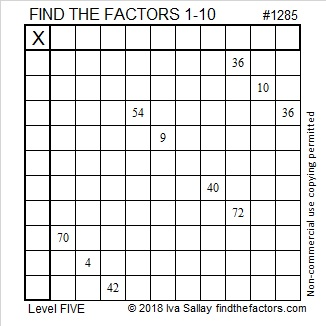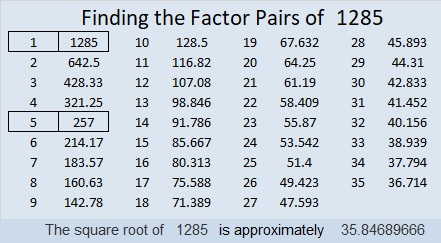# 1285 and Level 5

Level 5 puzzles can be tricky if you don’t carefully pick where you start, but you’re not going to let that discourage you, are you?Print the puzzles or type the solution in this excel file: 10-factors-1281-1288

Here are a few facts about the number 1285:

• 1285 is a composite number.
• Prime factorization: 1285 = 5 × 257
• The exponents in the prime factorization are 1 and 1. Adding one to each and multiplying we get (1 + 1)(1 + 1) = 2 × 2 = 4. Therefore 1285 has exactly 4 factors.
• Factors of 1285: 1, 5, 257, 1285
• Factor pairs: 1285 = 1 × 1285 or 5 × 257
• 1285 has no square factors that allow its square root to be simplified. √1285 ≈ 35.84695(2⁸ + 1) = 1285. Look at the digits on both sides of that equation. They are the reason that 1285 is the 20th Friedman number.

1285 is the sum of three consecutive prime numbers:
421 + 431 + 433 = 1285

1285 is the sum of two squares two different ways:
33² + 14² = 1285
31² + 18² = 1285

1285 is the hypotenuse of FOUR Pythagorean triples:
160-1275-1285 which is 5 times (32-255-257)
637-1116-1285 calculated from 31² – 18², 2(31)(18), 31² + 18²
771-1028-1285 which is (3-4-5) times 257
893-924-1285 calculated from 33² – 14², 2(33)(14), 33² + 14²

This site uses Akismet to reduce spam. Learn how your comment data is processed.